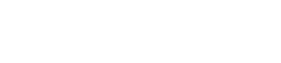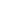## Mode Studio

The Collaborative Data Science Platform# SQL COUNT

Starting here? This lesson is part of a full-length tutorial in using SQL for Data Analysis. Check out the beginning.

## Counting all rows

`COUNT` is a SQL aggregate function for counting the number of rows in a particular column. `COUNT` is the easiest aggregate function to begin with because verifying your results is extremely simple. Let's begin by using `*` to select all rows from the Apple stock prices dataset:

``````SELECT COUNT(*)
FROM tutorial.aapl_historical_stock_price
``````

Note: Typing `COUNT(1)` has the same effect as `COUNT(*)`. Which one you use is a matter of personal preference.

You can see that the result showed a count of all rows to be 3555. To make sure that's right, turn off Mode's automatic limit by unchecking the box next to "Limit 100" next to the "Run" button in Mode's SQL Editor. Then run the following query:

``````SELECT * FROM tutorial.aapl_historical_stock_price
``````

Note that Mode actually provides a count of the total rows returned (above the results table), which should be the same as the result of using the `COUNT` function in the above query.

## Counting individual columns

Things start to get a little bit tricky when you want to count individual columns. The following code will provide a count of all of rows in which the `high` column is not null.

``````SELECT COUNT(high)
FROM tutorial.aapl_historical_stock_price
``````

You'll notice that this result is lower than what you got with `COUNT(*)`. That's because `high` has some nulls. In this case, we've deleted some data to make the lesson interesting, but analysts often run into naturally-occurring null rows.

For example, imagine you've got a table with one column showing email addresses for everyone you sent a marketing email to, and another column showing the date and time that each person opened the email. If someone didn't open the email, the date/time field would likely be null.

## Practice Problem

Write a query to count the number of non-null rows in the `low` column.

Try it out See the answer

## Counting non-numerical columns

One nice thing about `COUNT` is that you can use it on non-numerical columns:

``````SELECT COUNT(date)
FROM tutorial.aapl_historical_stock_price
``````

The above query returns the same result as the previous: 3555. It's hard to tell because each row has a different `date` value, but `COUNT` simply counts the total number of non-null rows, not the distinct values. Counting the number of distinct values in a column is discussed in a later tutorial.

You might have also noticed that the column header in the results just reads "count." We recommend naming your columns so that they make a little more sense to anyone else who views your work. As mentioned in an earlier lesson, it's best to use lower case letters and underscores. You can add column names (also called aliases) using `AS`:

``````SELECT COUNT(date) AS count_of_date
FROM tutorial.aapl_historical_stock_price
``````

If you must use spaces, you will need to use double quotes.

``````SELECT COUNT(date) AS "Count Of Date"
FROM tutorial.aapl_historical_stock_price
``````

Note: This is really the only place in which you'll ever want to use double quotes in SQL. Single quotes for everything else.

## Practice Problem

Write a query that determines counts of every single column. Which column has the most null values?

Try it out See the answer

Next Lesson

SQL SUM

Get the latest.